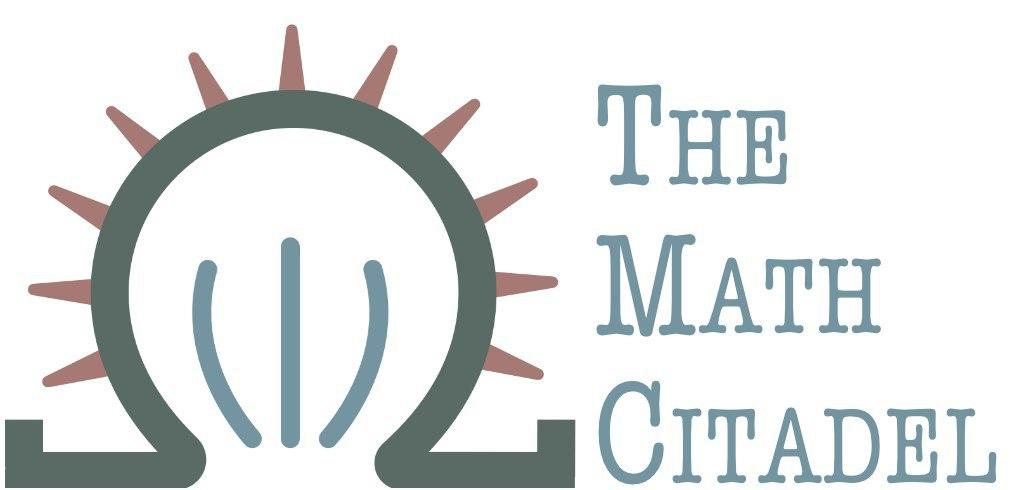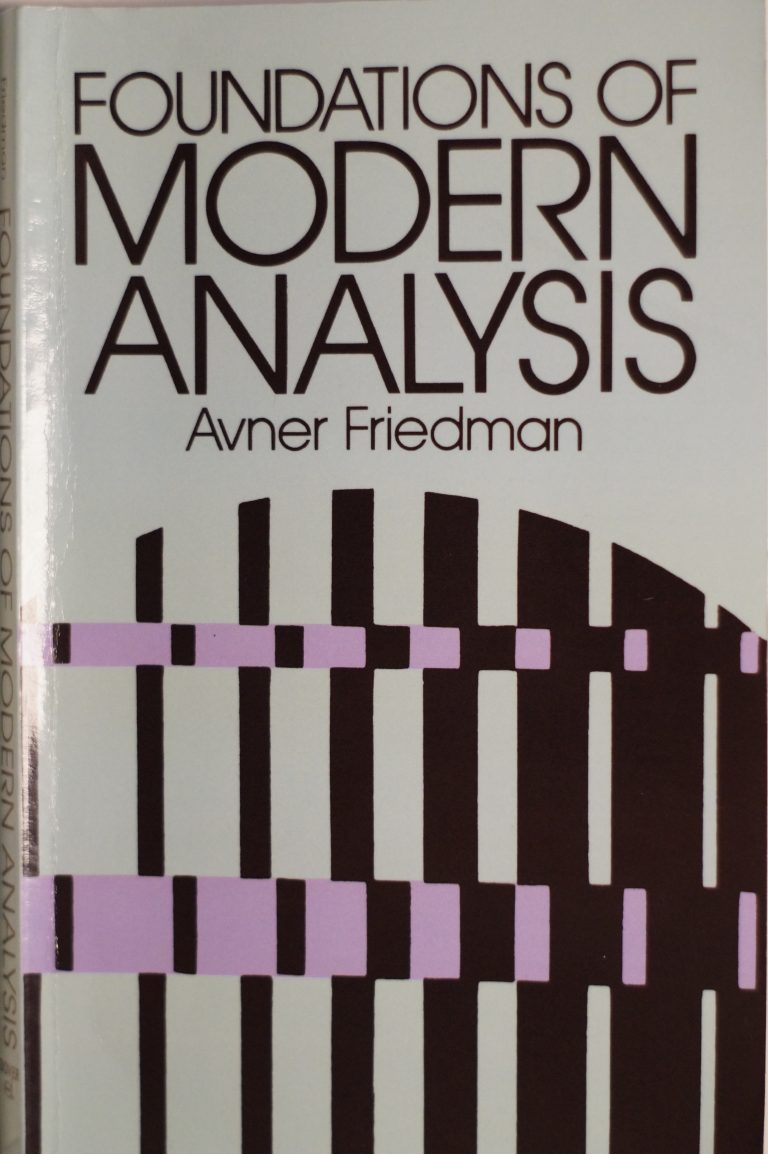## Book Review: Foundations of Modern Analysis

### Author: A. Friedman

#### Reviewed by: J. Hathcock## Ranking

All ranking is done on a scale of 1-5, with 5 as the best.
Attribute Rank
Difficulty 4
Proof Quality 2
Self-Study 2
Good for Teaching? 2
Quality of Exercises 2

## Topics Covered

• Measure Theory
• rings and algebras of sets
• inner and outer measure
• completion of measures
• Lebesgue and Lebesgue-Stieltjes measures
• metric outer measure
• signed measures
• Integration
• measurable functions
• Egoroff's Theorem
• convergence in measure
• integrals of simple functions
• definition and properties of integrals
• sequences of integrable functions
• Lebesgue's Bounded Convergence Theorem
• the Riemann integral
• The Radon-Nikodym Theorem
• Lebesgue decomposition
• product of measures
• Fubini's Theorem
• Metric Spaces
• Lp spaces
• completeness
• compactness
• continuous functions on metric spaces
• The Stone-Weierstrass Theorem
• fixed-points
• Banach Spaces
• normed linear spaces
• subspaces and bases
• linear transformations
• The Principle of Uniform Boundedness
• The Open-Mapping and Closed-Graph Theorems
• The Hahn-Banach Theorem
• conjugate and reflexive spaces
• Tychonoff's Theorem
• weak topology in conjugate spaces